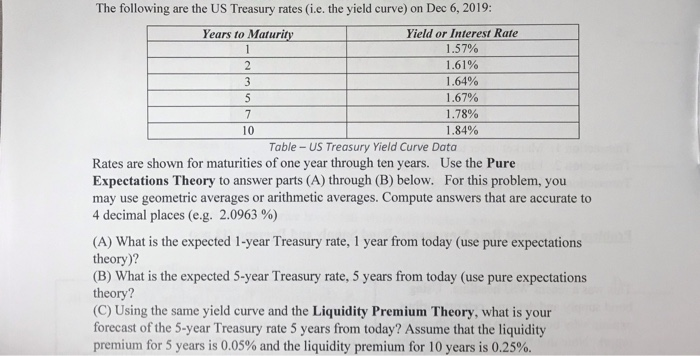### Create an Account

Home / Questions / 3 S 10 The following are the US Treasury rates the yield curve on Dec 6 2019 Years to M...

# 3 S 10 The following are the US Treasury rates the yield curve on Dec 6 2019 Years to Maturity Yield or Interest Rat 3 S 10 The following are the US Treasury rates3 S 10 The following are the US Treasury rates (i.e. the yield curve) on Dec 6, 2019: Years to Maturity Yield or Interest Rate 1.57% 2 1.61% 1.64% 1.67% 7 1.78% 1.84% Table - US Treasury Yield Curve Data Rates are shown for maturities of one year through ten years. Use the Pure Expectations Theory to answer parts (A) through (B) below. For this problem, you may use geometric averages or arithmetic averages. Compute answers that are accurate to 4 decimal places (e.g. 2.0963 %) (A) What is the expected 1-year Treasury rate, 1 year from today (use pure expectations theory)? (B) What is the expected 5-year Treasury rate, 5 years from today (use pure expectations theory? (C) Using the same yield curve and the Liquidity Premium Theory, what is your forecast of the 5-year Treasury rate 5 years from today? Assume that the liquidity premium for 5 years is 0.05% and the liquidity premium for 10 years is 0.25%.

Feb 08 2020 View more View LessGet Solution